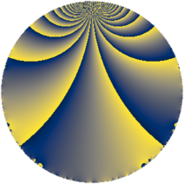Properties

 Label 108.2.hLevel $108$ Weight $2$ Character orbit 108.h Rep. character $\chi_{108}(35,\cdot)$ Character field $\Q(\zeta_{6})$ Dimension $8$ Newform subspaces $1$ Sturm bound $36$ Trace bound $0$

Related objects

Defining parameters

 Level: $$N$$ $$=$$ $$108 = 2^{2} \cdot 3^{3}$$ Weight: $$k$$ $$=$$ $$2$$ Character orbit: $$[\chi]$$ $$=$$ 108.h (of order $$6$$ and degree $$2$$) Character conductor: $$\operatorname{cond}(\chi)$$ $$=$$ $$36$$ Character field: $$\Q(\zeta_{6})$$ Newform subspaces: $$1$$ Sturm bound: $$36$$ Trace bound: $$0$$

Dimensions

The following table gives the dimensions of various subspaces of $$M_{2}(108, [\chi])$$.

Total New Old
Modular forms 48 16 32
Cusp forms 24 8 16
Eisenstein series 24 8 16

Trace form

 $$8 q + 3 q^{2} - q^{4} + 6 q^{5} + O(q^{10})$$ $$8 q + 3 q^{2} - q^{4} + 6 q^{5} - 8 q^{10} - 2 q^{13} - 12 q^{14} - q^{16} - 18 q^{20} + 3 q^{22} - 6 q^{25} - 12 q^{28} - 6 q^{29} + 33 q^{32} + 7 q^{34} - 8 q^{37} + 27 q^{38} + 10 q^{40} - 24 q^{41} + 12 q^{46} - 10 q^{49} - 21 q^{50} + 16 q^{52} - 18 q^{56} + 4 q^{58} - 2 q^{61} + 26 q^{64} + 30 q^{65} + 15 q^{68} - 6 q^{70} + 4 q^{73} + 30 q^{74} - 3 q^{76} + 30 q^{77} + 10 q^{82} + 8 q^{85} - 21 q^{86} - 21 q^{88} - 24 q^{92} - 18 q^{94} + 4 q^{97} + O(q^{100})$$

Decomposition of $$S_{2}^{\mathrm{new}}(108, [\chi])$$ into newform subspaces

Label Dim. $$A$$ Field CM Traces $q$-expansion
$a_{2}$ $a_{3}$ $a_{5}$ $a_{7}$
108.2.h.a $8$ $0.862$ 8.0.170772624.1 None $$3$$ $$0$$ $$6$$ $$0$$ $$q+\beta _{1}q^{2}+(-1+\beta _{1}+\beta _{3}-\beta _{5}-\beta _{7})q^{4}+\cdots$$

Decomposition of $$S_{2}^{\mathrm{old}}(108, [\chi])$$ into lower level spaces

$$S_{2}^{\mathrm{old}}(108, [\chi]) \cong$$ $$S_{2}^{\mathrm{new}}(36, [\chi])$$$$^{\oplus 2}$$